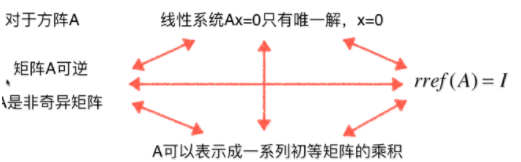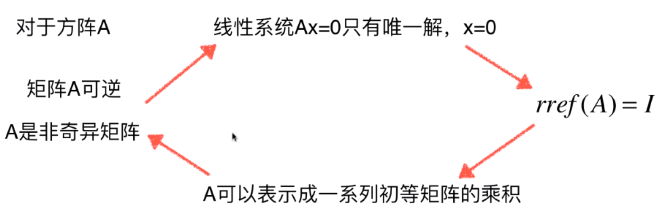• 初等矩阵性质
千次阅读
2021-07-01 22:37:13

初等矩阵性质：
1、设A是一个m×n矩阵，对A施行一次初等行变换，其结果等价于在A的左边乘以相应的m阶初等矩阵；对A施行一次初等列变换，其结果等价于在A的右边乘以相应的n阶初等矩阵。反之亦然。
2、方阵A可逆的充分必要条件是存在有限个初等矩阵P1，P2，…Pn，使得A=P1P2…Pn.
3、m×n矩阵A与B等价当且仅当存在m阶可逆矩阵P与n阶可逆矩阵Q使得B=PAQ。

更多相关内容
• 初等矩阵可逆初等矩阵：对单位矩阵 进行 一次 初等变换 得到。 初等矩阵一定是可逆的。 ==> 因为初等变换是可逆的，所以初等矩阵可逆的。 对于一般矩阵，如果可逆的话，怎样得到逆矩阵呢？ 根据之前的...

# 初等矩阵和可逆性

初等矩阵：对单位矩阵 进行 一次 初等变换 得到。

初等矩阵一定是可逆的。
==> 因为初等变换是可逆的，所以初等矩阵是可逆的。对于一般矩阵，如果可逆的话，怎样得到逆矩阵呢？
根据之前的分析 ==> 如果矩阵A可逆的话，即存在一系列的初等矩阵E，满足 ==>==> 在等式左右两端 乘以 A的逆==> 乘法结合律即矩阵A的逆 等于 对单位矩阵 进行 第一个初等变换，第二个初等变换 …

这个过程 等同于 ==>与使用线性系统的视角的求逆方法是一样的，只不过解决问题的视角不一样
之前将求解矩阵的逆的问题 转换成 一个求解线性系统的问题。
而这一次 将解矩阵的逆的问题 和 初等变换联系在了一起。

展开全文• 初等矩阵矩阵可逆性求解矩阵的逆矩阵的逆运算实现矩阵的逆 之前的笔记中曾提及过矩阵的逆的概念，但是并没有具体说明矩阵的逆的求法。 求解矩阵的逆 矩阵的逆运算 矩阵中AB=BA=IAB=BA=IAB=BA=I，则称B是A的逆矩阵...

### 初等矩阵和矩阵可逆性

之前的笔记中曾提及过矩阵的逆的概念，但是并没有具体说明矩阵的逆的求法。

# 求解矩阵的逆

## 矩阵的逆运算

矩阵中 A B = B A = I AB=BA=I ，则称B是A的逆矩阵，记为 B = A − 1 B=A^{-1} 只有方阵才有逆矩阵

求解逆矩阵以下面的例子作为引入，因为Gauss-Jordan消元法是针对系数矩阵进行操作，而结果矩阵是随着系数矩阵的变化而变化的，所以可以将增广矩阵进一步变化，
( 1 2 ∣ 1 0 3 4 ∣ 0 1 ) ⟹ ( 1 0 ∣ x 11 x 12 0 1 ∣ x 21 x 22 ) \begin{pmatrix}1&amp;2&amp;|&amp;1&amp;0\\3&amp;4&amp;|&amp;0&amp;1\end{pmatrix}\Longrightarrow\begin{pmatrix}1&amp;0&amp;|&amp;x_{11}&amp;x_{12}\\0&amp;1&amp;|&amp;x_{21}&amp;x_{22}\end{pmatrix}

左侧是系数矩阵，右侧是与之同形的单位矩阵，经过Gauss-Jordan消元法将左侧的系数矩阵化为行最简形式，如果化为行最简形式后左侧是一个单位矩阵，此时对应着唯一解的情况。上面的过程可以使用符号语言表示为，
( A ∣ I ) ⟹ ( I ∣ A − 1 ) \begin{pmatrix}&amp;A&amp;|&amp;I&amp;\end{pmatrix}\Longrightarrow\begin{pmatrix}&amp;I&amp;|&amp;A^{-1}&amp;\end{pmatrix}

矩阵的逆不可能存在无穷解，即一个方阵不可能存在无数个逆矩阵，只有存在一个逆矩阵或不存在逆矩阵这两种情况。

原因说明：
1.因为线性系统存在无穷解的情况可以看作是增广矩阵的最后一行为全0时对应的情况，但是求解逆矩阵时，增广矩阵右方是单位矩阵，不可能出现最下方的行为全0的情况。

2.当系数矩阵化为行最简形式后存在0行时，此时不存在逆矩阵。

上面的求解矩阵的逆的方式仅仅求取的是矩阵的右逆，与最开始矩阵的逆的定义相比，这看似是不严谨的。但是，另一个性质说明这种求取方式得到的结果是正确的，“如果一个方阵A有右逆B，则B也是A的左逆，即B是A的逆”。(以后给出证明)

## 实现矩阵的逆

求解矩阵的逆的过程本质上也是求解一个线性系统，只不过在一般的线性系统中，增广矩阵的右侧是一个列向量，而求逆时，增广矩阵的右侧是一个矩阵。所以依旧是在之前笔记的 LinearSystem类的基础上进行改造，

# -*- coding: utf-8 -*-
from .matrix import Matrix
from .vector import Vector
from ._global import EPSILON

class LinearSystem:
def __init__(self, A, b):
self._m = A.row_num()
self._n = A.col_num()
# 对构造方法进行如下修改
if isinstance(b, Vector):
self.Ab = [Vector(A.row_vector(i).underlying_list() + [b[i]]) for i in range(self._m)]
if isinstance(b, Matrix):
self.Ab = [Vector(A.row_vector(i).underlying_list() + b.row_vector(i).underlying_list() for i in range(self._m)]

self.pivots = []

def _forward(self):
......
def _backward(self):
......

# 对Gauss-Jordan消元法的返回值进行修改
def gauss_jordan_elimination(self):
self._forward()
self._backward()

# 检查系数矩阵为0时，结果矩阵不为0。此时是无解的情况
for i in range(len(self.pivots, self._m):
if not abs(self.Ab[i][-1]) < EPSILON:
return False
return True

def inv(A):
"""求解矩阵的逆"""
if A.row_num() != A.col_num():
return None
n = A.row_num()
ls = LinearSystem(A, Matrix.identity(n))
if not ls.gauss_jordan_elimination():
return None

invA = [[row[i] for i in range(n, 2*n)] for row in ls.Ab]

return Matrix(invA)



# 初等矩阵

## 矩阵的初等变换及初等矩阵

以该矩阵乘法进行说明，
( 1 0 0 0 1 0 0 0 1 ) ⋅ ( a b c d e f g h i ) = ( a b c d e f g h i ) \begin{pmatrix}1&amp;0&amp;0\\0&amp;1&amp;0\\0&amp;0&amp;1\end{pmatrix}\cdot \begin{pmatrix}a&amp;b&amp;c\\d&amp;e&amp;f\\g&amp;h&amp;i\end{pmatrix}= \begin{pmatrix}a&amp;b&amp;c\\d&amp;e&amp;f\\g&amp;h&amp;i\end{pmatrix}\\

1. 矩阵的某一行乘以一个常数
( k 0 0 0 1 0 0 0 1 ) ⋅ ( a b c d e f g h i ) = ( k ⋅ a k ⋅ b k ⋅ c d e f g h i ) \begin{pmatrix}k&amp;0&amp;0\\0&amp;1&amp;0\\0&amp;0&amp;1\end{pmatrix}\cdot \begin{pmatrix}a&amp;b&amp;c\\d&amp;e&amp;f\\g&amp;h&amp;i\end{pmatrix}= \begin{pmatrix}k\cdot a&amp;k\cdot b&amp;k\cdot c\\d&amp;e&amp;f\\g&amp;h&amp;i\end{pmatrix}
可总结为，
( k 0 0 0 1 0 0 0 1 ) \begin{pmatrix}k&amp;0&amp;0\\0&amp;1&amp;0\\0&amp;0&amp;1\end{pmatrix} 单位矩阵的某一行乘以一个常数。
2. 矩阵的一行加(减)另一行
( 1 0 0 0 1 0 1 0 1 ) ⋅ ( a b c d e f g h i ) = ( a b c d e f a + g b + h c + i ) \begin{pmatrix}1&amp;0&amp;0\\0&amp;1&amp;0\\1&amp;0&amp;1\end{pmatrix}\cdot \begin{pmatrix}a&amp;b&amp;c\\d&amp;e&amp;f\\g&amp;h&amp;i\end{pmatrix}= \begin{pmatrix}a&amp;b&amp;c\\d&amp;e&amp;f\\a+g&amp;b+h&amp;c+i\end{pmatrix}\\
可总结为，
( 1 0 0 0 1 − p 0 0 1 ) \begin{pmatrix}1&amp;0&amp;0\\0&amp;1&amp;-p\\0&amp;0&amp;1\end{pmatrix} 单位矩阵的一行加(减)另一行的若干倍。
3. 交换矩阵的两行
( 1 0 0 0 0 1 0 1 0 ) ⋅ ( a b c d e f g h i ) = ( a b c g h i d e f ) \begin{pmatrix}1&amp;0&amp;0\\0&amp;0&amp;1\\0&amp;1&amp;0\end{pmatrix}\cdot \begin{pmatrix}a&amp;b&amp;c\\d&amp;e&amp;f\\g&amp;h&amp;i\end{pmatrix}= \begin{pmatrix}a&amp;b&amp;c\\g&amp;h&amp;i\\d&amp;e&amp;f\end{pmatrix}\\
可总结为，
( 1 0 0 0 0 1 0 1 0 ) \begin{pmatrix}1&amp;0&amp;0\\0&amp;0&amp;1\\0&amp;1&amp;0\end{pmatrix} 交换单位矩阵的两行。

如上这3种操作统称为“矩阵的初等变换”，任何形式的初等变换都可以用相应形式的矩阵进行表示，而这些用于表示初等变换的矩阵称为“初等矩阵”，通常记为 E Ε

回顾之前的Gauss-Jordan消元法，把原矩阵化为行最简形式的过程实际上可以看作是寻找到一系列初等矩阵 E Ε ，使得
E p ⋅ . . . ⋅ E 3 ⋅ E 2 ⋅ E 1 ⋅ A = r r e f ( A ) E_p\cdot ...\cdot E_3\cdot E_2\cdot E_1\cdot A=rref(A)

## 初等矩阵和可逆性的关系

初等矩阵一定是可逆的

因为初等变换是可逆的，所以初等矩阵也是可逆的。对于前一小节的初等矩阵，其对应的逆矩阵形式如下，
( k 0 0 0 1 0 0 0 1 ) 逆矩阵 → ( 1 k 0 0 0 1 0 0 0 1 ) \begin{pmatrix}k&amp;0&amp;0\\0&amp;1&amp;0\\0&amp;0&amp;1\end{pmatrix}\underrightarrow{\text{逆矩阵}}\begin{pmatrix}\frac{1}{k}&amp;0&amp;0\\0&amp;1&amp;0\\0&amp;0&amp;1\end{pmatrix}
( 1 0 0 0 1 − p 0 0 1 ) 逆矩阵 → ( 1 0 0 0 1 p 0 0 1 ) \begin{pmatrix}1&amp;0&amp;0\\0&amp;1&amp;-p\\0&amp;0&amp;1\end{pmatrix}\underrightarrow{\text{逆矩阵}}\begin{pmatrix}1&amp;0&amp;0\\0&amp;1&amp;p\\0&amp;0&amp;1\end{pmatrix}
( 1 0 0 0 0 1 0 1 0 ) 逆矩阵 → ( 1 0 0 0 0 1 0 1 0 ) \begin{pmatrix}1&amp;0&amp;0\\0&amp;0&amp;1\\0&amp;1&amp;0\end{pmatrix}\underrightarrow{\text{逆矩阵}}\begin{pmatrix}1&amp;0&amp;0\\0&amp;0&amp;1\\0&amp;1&amp;0\end{pmatrix}
回顾Gauss-Jordan消元过程求取矩阵的逆，
E p ⋅ . . . ⋅ E 3 ⋅ E 2 ⋅ E 1 ⋅ A = r r e f ( A ) = I E_p\cdot ...\cdot E_3\cdot E_2\cdot E_1\cdot A=rref(A)=I
如果矩阵 A A 可逆，则存在一系列初等矩阵 E E ，使得
E p ⋅ . . . ⋅ E 3 ⋅ E 2 ⋅ E 1 ⋅ A ⋅ A − 1 = I ⋅ A − 1 E_p\cdot ...\cdot E_3\cdot E_2\cdot E_1\cdot A\cdot A^{-1}=I\cdot A^{-1}
E p ⋅ . . . ⋅ E 3 ⋅ E 2 ⋅ E 1 ⋅ I = A − 1 E_p\cdot ...\cdot E_3\cdot E_2\cdot E_1\cdot I=A^{-1}
即，
( A ∣ I ) ⟹ ( I ∣ A − 1 ) \begin{pmatrix}&amp;A&amp;|&amp;I&amp;\end{pmatrix}\Longrightarrow\begin{pmatrix}&amp;I&amp;|&amp;A^{-1}&amp;\end{pmatrix}

# 矩阵可逆的重要性

## 加速线性系统计算

对于线性系统 A x = b Ax=b ，有如下推导，
A − 1 ⋅ A ⋅ x = A − 1 ⋅ b x = A − 1 ⋅ b A^{-1}\cdot A\cdot x=A^{-1}\cdot b\\x=A^{-1}\cdot b
当线性系统的系数矩阵A不变，b会变化的情况下，使用矩阵的逆进行计算会大大加快计算速度。

## 矩阵的逆相关重要命题

矩阵的逆与很多重要的命题相关，这一系列的命题都是等价对于上面诸多问题的证明，逐一证明时间太长，最好的方式是将四个命题串成串来证明，如顺时针方向证明各个命题，

1. 证明：对于方阵 A A ，若 A A 可逆（是非奇异矩阵），则当线性系统 A x = 0 Ax=0 时，仅有唯一解 x = 0 x=0
A ⋅ x = 0 A\cdot x=0
两端同乘 A − 1 A^{-1} ，得到
A − 1 ⋅ A ⋅ x = A − 1 ⋅ 0 I ⋅ x = 0 x = 0 A^{-1}\cdot A\cdot x=A^{-1}\cdot0\\I\cdot x=0\\x=0
2. 证明：若线性系统 A x = 0 Ax=0 只有唯一的0解，则 A A 的行最简形式 r r e f ( A ) = I rref(A)=I
因为 A A 是n*n的矩阵，且 A x = 0 Ax=0 有唯一解，则有 n n 个未知数，且 r e f f ( A ) reff(A) 存在 n n 个非0行
根据行最简形式的定义： r r e f ( A ) = I rref(A)=I
3. 证明：若矩阵 A A 的行最简形式 r r e f ( A ) = I rref(A)=I ，则 A A 可以表示成一系列初等矩阵的乘积
依据上面的表述可写出，
E p ⋅ . . . ⋅ E 3 ⋅ E 2 ⋅ E 1 ⋅ A = r r e f ( A ) = I E_p\cdot ...\cdot E_3\cdot E_2\cdot E_1\cdot A=rref(A)=I
等式两端同乘一连串逆矩阵，
E 1 − 1 ⋅ E 2 − 1 ⋅ E 3 − 1 ⋅ . . . ⋅ E p − 1 ⋅ E p ⋅ . . . ⋅ E 3 ⋅ E 2 ⋅ E 1 ⋅ A = E 1 − 1 ⋅ E 2 − 1 ⋅ E 3 − 1 ⋅ . . . ⋅ E p − 1 ⋅ I E_1^{-1}\cdot E_2^{-1}\cdot E_3^{-1}\cdot ...\cdot E_p^{-1}\cdot E_p\cdot ...\cdot E_3\cdot E_2\cdot E_1\cdot A=E_1^{-1}\cdot E_2^{-1}\cdot E_3^{-1}\cdot ...\cdot E_p^{-1}\cdot I
可得，
A = E 1 − 1 ⋅ E 2 − 1 ⋅ E 3 − 1 ⋅ . . . ⋅ E p − 1 A=E_1^{-1}\cdot E_2^{-1}\cdot E_3^{-1}\cdot ...\cdot E_p^{-1}
4. 证明：若矩阵 A A 可以表示成一系列初等矩阵的乘积，则该矩阵可逆（是非奇异矩阵）
A = E 1 ⋅ E 2 ⋅ E 3 ⋅ . . . ⋅ E p A=E_1\cdot E_2\cdot E_3\cdot ...\cdot E_p
假设存在一个矩阵 B B ，满足
B = E p − 1 ⋅ . . . ⋅ E 3 − 1 ⋅ E 2 − 1 ⋅ E 1 − 1 B=E_p^{-1}\cdot ...\cdot E_3^{-1}\cdot E_2^{-1}\cdot E_1^{-1}
有矩阵的逆的运算性质， B B 可以表示为，
B = ( E 1 ⋅ E 2 ⋅ E 3 ⋅ . . . ⋅ E p ) − 1 B=(E_1\cdot E_2\cdot E_3\cdot ...\cdot E_p)^{-1}
计算可得，
A ⋅ B = I B ⋅ A = I A\cdot B=I\\B\cdot A=I
故， A A 是可逆矩阵，其逆矩阵为 B B

由此整个环证明完成，随便抽取出两个命题，都可以间接地通过环得出这两个命题是等价的。同时这四个命题的否命题也是等价的。

所谓两个事物“等价”可以很简单的理解为，说其中一件就等同于说另一件。在上面的四个命题中，提及矩阵可逆，则等同于又说了其他三个命题。

展开全文• ## 可逆矩阵的性质

万次阅读 多人点赞 2018-07-19 09:01:44
在人工智能的基础学习中，...2 可逆矩阵一定是方阵。 3 如果矩阵A是可逆的，A的逆矩阵是唯一的。 4 可逆矩阵也被称为非奇异矩阵、满秩矩阵。 5 两个可逆矩阵的乘积依然可逆。 6 可逆矩阵的转置矩阵也可逆。 7 ...
在人工智能的基础学习中，经常会用到矩阵相关的问题，这里假设A为n*n阶矩阵，则记录了A矩阵可逆与不可逆的一些性质，希望对大家有帮助：

A可逆的性质：
1 矩阵A可逆的充要条件是A的行列式不等于0。
2 可逆矩阵一定是方阵。
3 如果矩阵A是可逆的，A的逆矩阵是唯一的。
4 可逆矩阵也被称为非奇异矩阵、满秩矩阵。
5 两个可逆矩阵的乘积依然可逆。
6 可逆矩阵的转置矩阵也可逆。
7 矩阵可逆当且仅当它是满秩矩阵。
A矩阵不可逆
1 |A| = 0
2 A的列(行)向量组线性相关
3 R(A)<n
4 AX=0 有非零解
5 A有特征值0.
6 A不能表示成初等矩阵的乘积
7 A的等价标准形不是单位矩阵
展开全文• 可逆矩阵（定义、充要条件、与初等矩阵）、分块矩阵相似对角化、正交矩阵（定义、充要条件及性质
• 文章目录1 线性系统与矩阵的逆2 初等矩阵3 从初等矩阵矩阵的逆4 为什么矩阵的逆这么重要5 矩阵的LU分解6 非方阵的LU分解，矩阵的LDU分解和PLU分解7 矩阵的PLUP分解和再看矩阵的乘法参考资料 注：转载请标明原文...
• 可逆矩阵，又称非奇异矩阵，其逆矩阵唯一 AAA可逆⇔∣A∣≠0\Leftrightarrow |A|\ne0⇔∣A∤​=0且A−1=A∗∣A∣A^{-1}=\frac{A^*}{|A|}A−1=∣A∣A∗​其中A∗A^*A∗是伴随矩阵，由一系列代数余子式（分正负号的...
• 展开全部n阶方阵矩阵可逆，则|A|≠0，即e5a48de588b63231313335323631343130323136353331333366303838|A|是A的n阶非零子式，...若方阵的逆阵存在，则称为可逆矩阵或非奇异矩阵，且其逆矩阵唯一。设A是n阶矩阵, 若r(A...
• 文章目录矩阵分块法常用的分块法1) 按行分块2) 按列分块3) 分块对角矩阵(又称准对角矩阵)分块矩阵的运算分块矩阵初等变换分块初等矩阵性质参考 矩阵分块法 定义1\large\color{magenta}{\boxed{\color{brown}{...自然语言处理 深度学习 数据挖掘 机器学习
• 二、可逆矩阵 定理一： 证明该定理： * * * 单位矩阵恒等变形 * * * 定理二： 证明： 把 读成B，就是AB = BA = E，就成了矩阵可逆的定义。 几个小公式： 二、计算可逆矩阵 1、通过伴随矩阵来求 2、 ...
• 本节为线性代数复习笔记的第二部分，矩阵的概念与计算（1），主要包括：行列式的几何意义，行列式的展开计算（余子式，代数余子式），行列式的性质，特殊的五个行列式以及克拉默法则。 1. 欢迎扫描二维码关注微信...
• 矩阵的逆1.1 逆矩阵的定义1.2 逆矩阵性质与重要公式1.3 用定义求逆矩阵1.4 例题2. 伴随矩阵2.1 伴随矩阵的定义2.2 伴随矩阵的定义与重要公式2.3 用伴随矩阵求逆矩阵3. 转置、伴随、逆矩阵、取行列式公式小结3.1 ...
• 初等矩阵矩阵可逆矩阵的LU分解概念LU分解过程LU分解的意义代码实现 矩阵的LU分解 概念 矩阵的分解实际上就是将一个矩阵分解成为几个矩阵乘积的形式。不同类型的矩阵分解有不同的目的，如矩阵的LU分解的目的是...
• 第三章，矩阵，06-初等变换与初等矩阵标准形初等行变换初等列变换初等变换行阶梯形矩阵行最简形标准形初等矩阵定义性质可逆等价矩阵相关定义行等价列等价等价性质定理1定理2推论 玩转线性代数(18)初等变换与初等矩阵...
• 求解矩阵的逆： 接着https://www.cnblogs.com/webor2006/p/14280299.html继续往下学习，在上一次中学习了线性系统以及它的求解， 在之前https://www.cnblogs.com/webor2006/p/14271706.html的学习矩阵的逆时遗留了一...机器学习 人工智能 webgl 编程语言
• 之前介绍了如何将一个矩阵化成上三角矩阵，并且提到了矩阵的秩可以通过上三角矩阵轻松得出，秩就是上三角矩阵中非零行的个数，不知道怎么将一个矩阵通过初等行变换的伙伴们可以参考我的上一篇文章 ...c++ 机器学习
• 而秩是矩阵的一个特性，他是矩阵是否有可逆矩阵的标志。 1 初等行变换的种类 1.1 两行交换 1.2 某行乘以非零常数 1.3 某行乘以常数加到另外的一个行 2 初等行变换求逆 加一个单位矩阵，然后，变化成单位...
• ## 可逆矩阵的特征

千次阅读 2019-04-23 23:15:49
定理 7 n*n矩阵A是可逆的，当且仅当A行...a A是可逆矩阵 b A等价于nn单位矩阵 c A有n个主元位置 d 方程Ax=0仅有平凡解 e A的各列线性无关 f 线性变化x->Ax是一对一的 g 对Rn中任意b，方程Ax=b至少有一个解 h A...
• 如果存在使得，则A是可逆的、非奇异的，且使得=。 逆矩阵的概念类似于数学中的导数，5*(1/5)=1，，矩阵A的“倒数”是它的逆矩阵。 逆矩阵产生的原因： 用来实现矩阵的除法。比如有矩阵X，A，B，其中X*A = B，...
• 矩阵初等变换 初等行变换： 对换两行，对换i，j 两行，记作：ri↔rjr_i \harr r_j ri​↔rj​ 以一个不等于 k 的数乘某一行中的所有元，第 i 行乘与 k 记作：ri×kr_i \times kri​×k 把某一行的 n 倍加到另一行...
• 思路：由于p为任意可逆矩阵，范围较大不便于研究，我们可从最简单的可逆矩阵E（i，j），E（i（k）），E（i，j（k））入手（下面证明只需用前两种初等矩阵） 从初等矩阵入手，是因为任意可逆矩阵p都能化为一些初等...
• 分块矩阵初等变换，以及简单证明；对于主次对角线分块矩阵、四分块矩阵、上下三角分块矩阵的行列式与逆的快速求法
• ## 矩阵的初等变换

千次阅读 2021-12-22 19:11:57
矩阵初等变换1.方程组的每一个方程进行变换:2.矩阵初等变换：计算逆矩阵，如果按照 逆矩阵等于：行列式A的倒数乘以伴随矩阵，就计算量有点大。所以可以采用定理13.矩阵的秩： 1.方程组的每一个方程进行变换: 1和2...机器学习
• 应对n这个变量，可以使用矩阵的初等变换来求解矩阵的可逆矩阵 矩阵的初等变换 1） 消元法解线性方程组 先来看下这个例子，从x1∼x4x_1 \sim x_4x1​∼x4​ 和 4个方程，求解线性方程组 有这样一个方程组 {2x1−x2...AI 数学
• ## 2.6 矩阵的初等变换

千次阅读 2020-01-08 13:26:10
初等变换的本质是：对矩阵的变化 只有当矩阵A为方阵时，初等变换才会和行列式产生联系 定理 任何矩阵都能通过初等变换化为标准型 化为标准型的处理过程为： 先处理第一列 等价 性质 反身性、对称性、传递性、...
• ## 可逆矩阵

千次阅读 2019-11-13 18:27:08
方阵满秩时，可以使用初等行变换，化成单位矩阵（相当于使用一系列初等矩阵左乘矩阵，得到单位矩阵），从而可逆 行满秩矩阵就是行向量线性无关，列满秩矩阵就是列向量线性无关 例：判断矩阵AAA是否行满秩 ...
• 3、每一个(λ-λi)ri对应一个若当块Ji，其中i=1,2,…,q，ri阶方阵Ji为： 4、矩阵A的若当标准型J为： 5、一定存在n阶可逆矩阵P，使得P-1AP=J。将n阶可逆方阵P分块为P=[P1,P2,…,Pq]，其中n×ri阶矩阵Pi=[pi1,pi2,…,...Jordan标准型 行列式因子 不变因子 Smith标准型
• 文章目录矩阵与复数逆矩阵的定义可逆矩阵的逆矩阵的求法可逆矩阵性质克拉默法则的另一种推导法矩阵乘积的秩的性质参考 矩阵与复数 复数可以用二维有序数组来表示，如复数 a+bia+b \mathrm{i}a+bi 可表示为 (a,b),...自然语言处理 数据挖掘 机器学习 深度学习
• ## 矩阵的行初等变换

千次阅读 多人点赞 2019-08-13 10:51:09
介绍了行初等变换的概念；实现方式；行初等变换矩阵的行列式；以及经过行初等变换矩阵的行列式；...